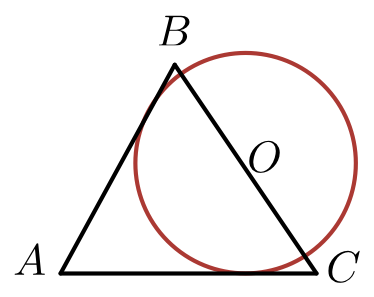# The Hat Problem

Geometry Level 4Circle $O$ is drawn on plane $K$. On the same plane, $\Delta ABC$ with $AB=13$, $AC=14$, and $BC=15$ is drawn such that $AB$ and $AC$ are tangents to the circle at distinct points, and $BC$ contains the center $O$. If the area of circle $O$ can be expressed in the form $\frac{a\pi}{b}$, where $a$ and $b$ are positive coprime integers, find the value of $a+b$.

Draw a smiley face using the circle, and the title is justified. :)

×

Problem Loading...

Note Loading...

Set Loading...